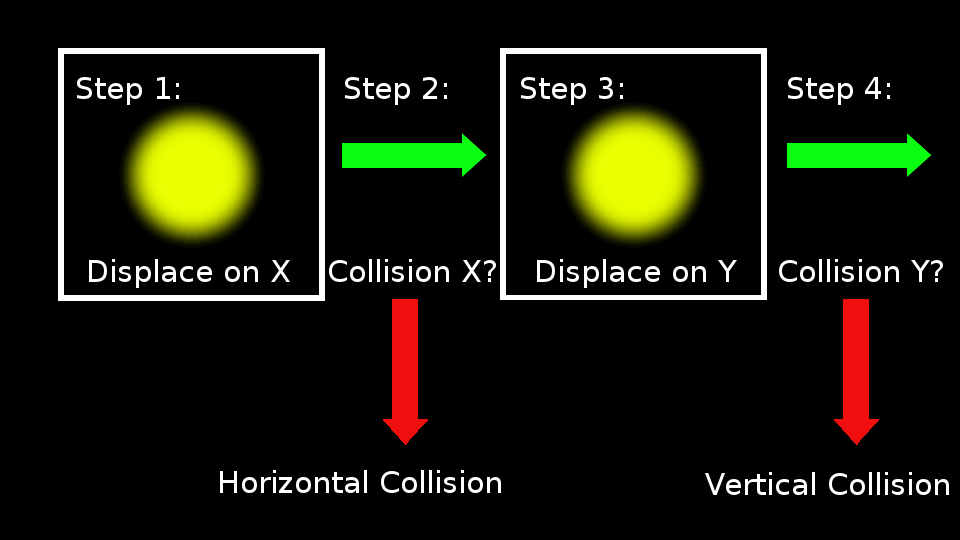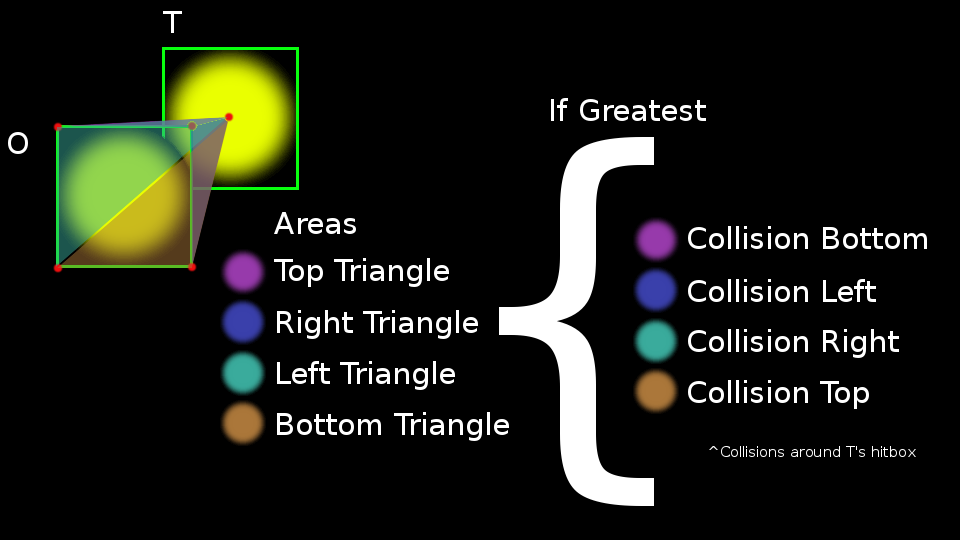Collision Orientation Detection

This is a summary of various models I have used for determining the direction a collision has occurred in without using preemptive checks. I have no need to actually perform these tasks in my game, and I noticed a lot of different models online that did not ever help me resolve this issue knowing my title did not need to assess potential collisions ahead of time.

Posted by on

I hope this is beneficial to anyone, anywhere, looking to resolve collision orientations. Two days ago I finalized my collision system to use a higher precision model that would detail quite well which direction a collision was occurring in when referenced to one other object. I had started out with a low precision model that I based my goals around, using this helped to actually derive the method used now. If you do not care about why I have my own model and what happened to build it, skip to the end, I will bold the proof I made.

Firstly, everyone seems to love this method; but this is a method used for certain types of games. Move one axis at a time and in between do a collision test. Quite simple, no need to worry about left or top bounds occurring first. Straight cut and dry. The movement I am using cannot use this method in an ideal way, and it would cut out some details that would be quite important in observation. The style of game where this would work is one where any object moving can only move at the same rate both vertically and horizontally. This is by far the most popular thing I found online, but does still feature something I like to call Problem of Equal Proportions.Problem of Equal Proportions is a collision occurring wherein both hitboxes meet at even distributions from a corner. This is always going to come up as a potential threat to any method of collision direction tests, and the easiest thing to do is have a resolve in place to catch it. The above method of one displacement at a time resolves it by favoring the direction first tested to have a collision occur.

Knowing I was not able to use the most popular method I moved onto a weighted system. The first method would not work due to having potential for unequal X and Y based vectors(from here on vX, vY), meaning an X collision might occur in a frame, but a Y collision might occur too if its potential motion(vY) is greater than vX or if vX is greater than vY but their lengths(delta: dX, dY) differ where vX at dX causes a collision and the lesser vY but at a closer dY also cause a collision. The weighted system was funky at best. I found an interesting relationship using trigonometry, but it was inaccurate enough to cause worry, but also required trig look-ups which are potentially detrimental to overall performance.

The first weighted system I used was basing a physical presence over another physical presence. More specifically an area of a triangle was compared to another triangular area. The basis of this was completely fabricated from pen and paper relationship tests. The idea was similar to, "Maybe the greater or lesser length of an overlap on an axis suggests which collided first," which is completely valid idea but wrong on its own by just using a measurement because the vX and vY could differ. Sure enough measuring area between two objects actually gave me something quite interesting. Setting an object as a target(T) and another objectionable object(O) to compare to T, I was able to measure varying triangle areas between the two to result in a better estimate -- than just overlap lengths -- of which direction T hit O first. I calculate four areas of four different triangles. The triangles were made by connecting T's center hitbox point to two other vertices taken from the four corners of O's hitbox. More often than not I found the largest area was the opposite side of where the collision occurred. So if an area of a triangle was found using the top left and bottom left points of O's hitbox connected then to the center of T's hitbox, and the area of this triangle was greater than the areas found combining the other edge corners of O's hitbox, then it suggests that T collided on O's right side. This was cool, while completely impractical. Its coolness is based on not needing to run conditions based on the actual direction of T's vX and vY, and how it is relative to O's vX and vY. The impractical state is based on needing to actually perform trig look-ups and use square roots to find the areas of these triangles; not to mention it was also inaccurate.This made way to better values to pay attention to when weighting, but most importantly to go ahead and heed the direction attached to the vectors. Doing so greatly avoids using any trigonometric lookup. While nifty, looking up these values does kill useful processing time. The next procedure greatly reduced this risk, but was still an approximation mostly to just be able to test mechanics more than results of collision directions. At this point I had moved onto performing collisions to push objects around to lead into more dynamic gameplay elements later. So I mostly just used something that was fast but not definite.

The next stage was of taking the weight of T's vectors, more specifically defining T as having a rate, and describing vX and vY as two fractions that make a whole value of one when if added. So, the rate was distributed between weights of an object's vector. I observed the sign of vX and vY to determine which direction the object is traveling in global coordinate space. In my method for determining the hit direction I added conditions based on these signs to determine what side a collision ultimately occurred on after resolving whether it was from a vertical or horizontal side first. I found dX and dY by looking at the hitbox boundaries of T and O and determining which corner of T overlapped O in favor of the direction T approached from based on the sign of T's vX and vY. Here is a where the approximation comes in. I took a relative weight of vX and vY(from here on vRX and vRY) by removing O's vX and vY fractional weights from T's vX and vY fractional weights. Then I found a value for X and Y to determine whether the collision was vertical or horizontal: X = vRX / dX and Y = vRY / dY. This math is gross, but it gave approximate results to further my testing of dynamism. It does not base the differing rates between T and O when comparing weighted vectors vX and vY which are of course prudent when actually making a relative comparison. As I have finished testing the dynamic results of my collision system and left open areas to plug and play new details based on this system, I decided it was well worth the time to make a new procedure.

The previous method was figured on the fly to continue collision testing operations, and having time to sit on it I came up with an elegant simple result, add the rate information and base results on time. The weights of vX and vY are still useful elsewhere, so I continue to use them in this method, but I convert them to actual magnitudes of the vector for both T and O instead of leaving them in a weighted form for the rates of T and O. I make one relative value that is accurate, with this accuracy I can avoid tedious condition checks to determine dX and dY of a collision length between T and O, and then I can use vRX and vRY to find the amount of time it would have taken to travel along dX at vRX and dY at vRY. That is time(tX and tY) is tX = dX / vRX and tY = dY / vRY. The collision occurred on the axis with the least amount of time. This is because my collisions are based on having a measurable area in the collision, that is both dX and dY have to be greater than zero. A longer time suggests that one axis, say X, had an intersection occur on that axis, X, before an intersection also occurred on the other axis, Y. Without both axis intersecting between T and O no collision is considered to have occurred. Using this I still of course need a fallback to "Problems of Equal Proportions", meeting up at a corner, but also introduces one other condition to check, whether or not a relative velocity, vRX or vRY, is equal to zero lest I create a hole in the game world when solving for time.

So that is it. I hope that helps someone at some point when they are making a game where rates and scales are in favor of testing collision boundaries only by the information given in the frame where they occur as opposed to checking preemptive collision possibilities from a frame before a collision. For those of you who want to get the jist of what my current method of direction testing is, and you jumped through the write-up to get here then here is a proof: having vectors for objects separated by a x vector(vX) and a y vector(vY), where vX + vY = actual vector, then adding a target's(T) and another object's(O) separated vectors together to make separated relative vectors(vRX and vRY), where vRX = T's vX + O's vX and vRY = T's vY + O's vY, and using the appropriate deltas -- for x(dX) and y(dY) -- of an overlap between a T and O based on the relative vectors, then if neither of the relative vectors is equal to zero the amount of time -- for x(tX) and y(tY) -- needed to travel on along dX by vRX and dY by vRY can be found, where tX = dX / vRX and tY = dY / vRY. Assuming a collision is considered to occur only if both dX and dY are greater than zero, then the time of shortest length determines the axis where the collision first occurred, as with only one delta being greater than zero a collision has occurred with one axis, but does not validate that an overlap with another object has occurred meaning that a greater time suggests T crossed the boundary of O on a single axis before an actual collision occurred. Once the axis of the collision is determined, observing the direction attached to the corresponding relative vector results in the direction that T hit O(if negative vR(X||Y) then the negative side of the object in accordance to the coordinate space else positive). So, rVX = T.vX + O.vX and rVY = T.vY + O.vY; tX = dX / rVX and tY = dY / rVY; if tX < tY then collision on X axis, if tY < tX then collision on Y axis, if tX == tY dispute and pick an axis; if rV(X for X axis or Y for Y axis) is negative then side of the axis where the collision occurred is the more negative one, else it is the more positive one.